## RS Aggarwal Class 8 Solutions Chapter 3 Squares and Square Roots Ex 3F

These Solutions are part of RS Aggarwal Solutions Class 8. Here we have given RS Aggarwal Solutions Class 8 Chapter 3 Squares and Square Roots Ex 3F.

Other Exercises

Evaluate:

Question 1.
Solution: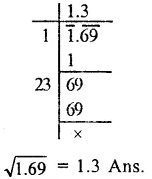Question 2.
Solution: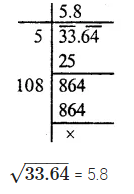$$\sqrt { 33.64 }$$ = 5.8

Question 3.
Solution: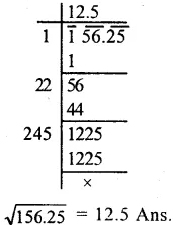Question 4.
Solution:Question 5.
Solution:Question 6.
Solution: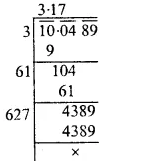$$\sqrt { 10.0489 }$$ = 3.17

Question 7.
Solution: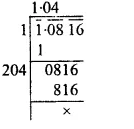$$\sqrt { 1.0816 }$$ = 1.04

Question 8.
Solution: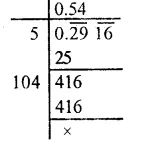$$\sqrt { 0.2916 }$$ = 0.54

Question 9.
Solution:
$$\sqrt { 3 }$$ = 1.73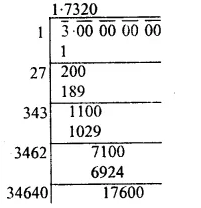Question 10.
Solution:
$$\sqrt { 2.8 }$$ = 1.6733 = 1.67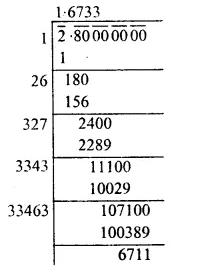Question 11.
Solution:
$$\sqrt { 0.9 }$$ = 0.948
= 0.95Question 12.
Solution:
Length of rectangle (l) = 13.6 m
and width (b) = 3.4 m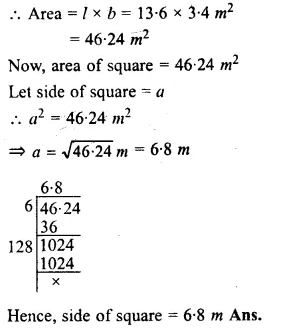Hope given RS Aggarwal Solutions Class 8 Chapter 3 Squares and Square Roots Ex 3F are helpful to complete your math homework.

If you have any doubts, please comment below. Learn Insta try to provide online math tutoring for you.